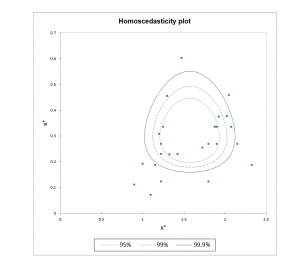# Inter-laboratory proficiency testing

Use this tool to evaluate and compare the measuring results obtained by different laboratories. Available in Excel using the XLSTAT software.## What is inter-laboratory proficiency testing?

Proficiency testing, also called interlaboratory comparison, involves using statistical methods to compare the performance of several participants (which may be laboratories, inspection bodies, or individuals), referred to as “items” in XLSTAT, for specific measurements (referred to as “tests” in XLSTAT). Proficiency testing can be performed to assess the performance of laboratories making measurements, to detect problems in one or more laboratories when they arise, or to establish effectiveness and comparability of different methods.

The method consists of identifying, then removing or ignoring outliers and producing robust estimates of both location and scale estimators.

This tool is based on the ISO-13528 standard. It was first developed using the 2015 edition. As some errors have been detected in the 2015 version of the document (see here further details), XLSTAT gives you the possibility to run the analysis with or without the errors in the computations of the Qn scale estimate.

## Options for inter-laboratory proficiency testing in XLSTAT

XLSTAT allows for a highly automated analysis of the laboratory data and can yield a series of general and robust statistics that can then be used to interpret the results and define to what extent the proficiency standards are met. While many of the XLSTAT functions can be used to analyze inter-laboratory data, the automation provided here is very useful when the user wants to use recommended but more complex algorithms, such as Algorithm A, Algorithm S or the Q/Hampel approach.

The Circle Technique (Van Nuland, 1992) is a very effective and underused technique to simultaneously compare the means and the variances of several items, while allowing you to identify potential outliers.

Algorithm A and the Q/Hampel method are used to obtain robust estimators of mean and standard deviation. Algorithm S is used to estimate the scale estimator from standard deviations or ranges.

## Results for inter-laboratory proficiency testing in XLSTAT

XLSTAT displays several tables and a chart to help in analyzing and interpreting the results.

If there are multiple items (laboratories) and if two or more tests (measurements) have been performed for each of them, XLSTAT displays a list of summary statistics for each of them, including robust statistics.

Summary statistics are then computed across all items.

If possible, the homoscedasticity plot is displayed to compare the location (horizontal axis) and scale (vertical axis) of the different items (e.g laboratories). The greater the spread on the vertical axis is, the less valid is the assumption of constant variance across the items. Confidence lines (90%, 95%, 99%) are displayed to identify items that show values that are potential outliers, such as laboratories which do not meet the standards.

Last, if there are multiple items (laboratories) and if two or more tests (measurements) have been performed, XLSTAT displays the results of the advanced algorithms described in ISO-13528 (Algorithm A, Algorithm S, Q/Hampel) that are designed to compute location and scale estimators iteratively. Algorithm A and the Q/Hampel method are used to obtain robust estimators of scale and standard deviation. Algorithm S is used to estimate the scale estimator from standard deviations or ranges.### analyze your data with xlstat

14-day free trial

Included in

Related features